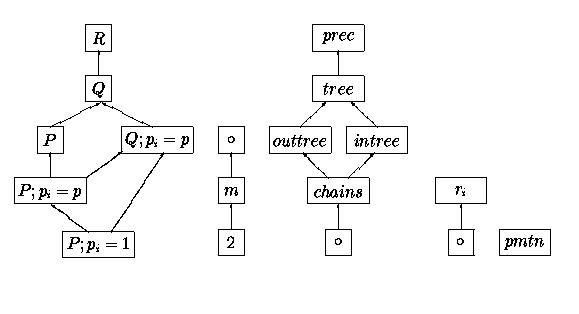# Parallel machine problems with preemptionIn the case of uniform machines we assume that the machine speeds are rational numbers. Then problems with unit processing times are equivalent to problems with constant processing times pi=p, i.e. we do not consider the case pi=1 for uniform machines Q.